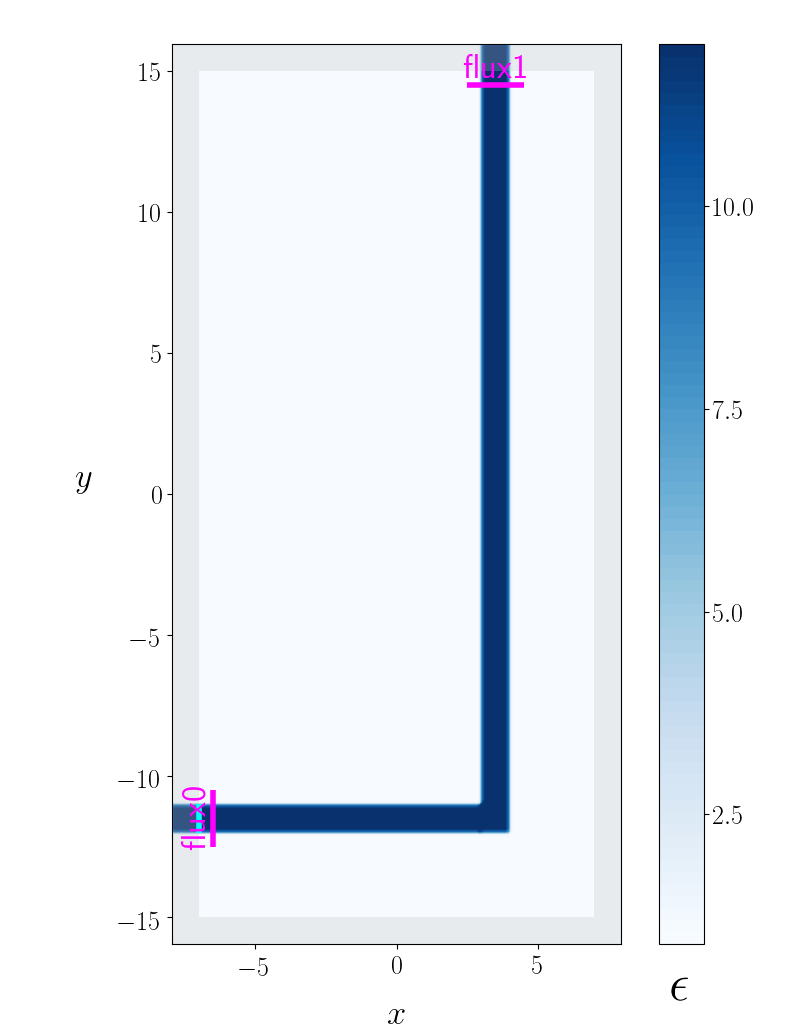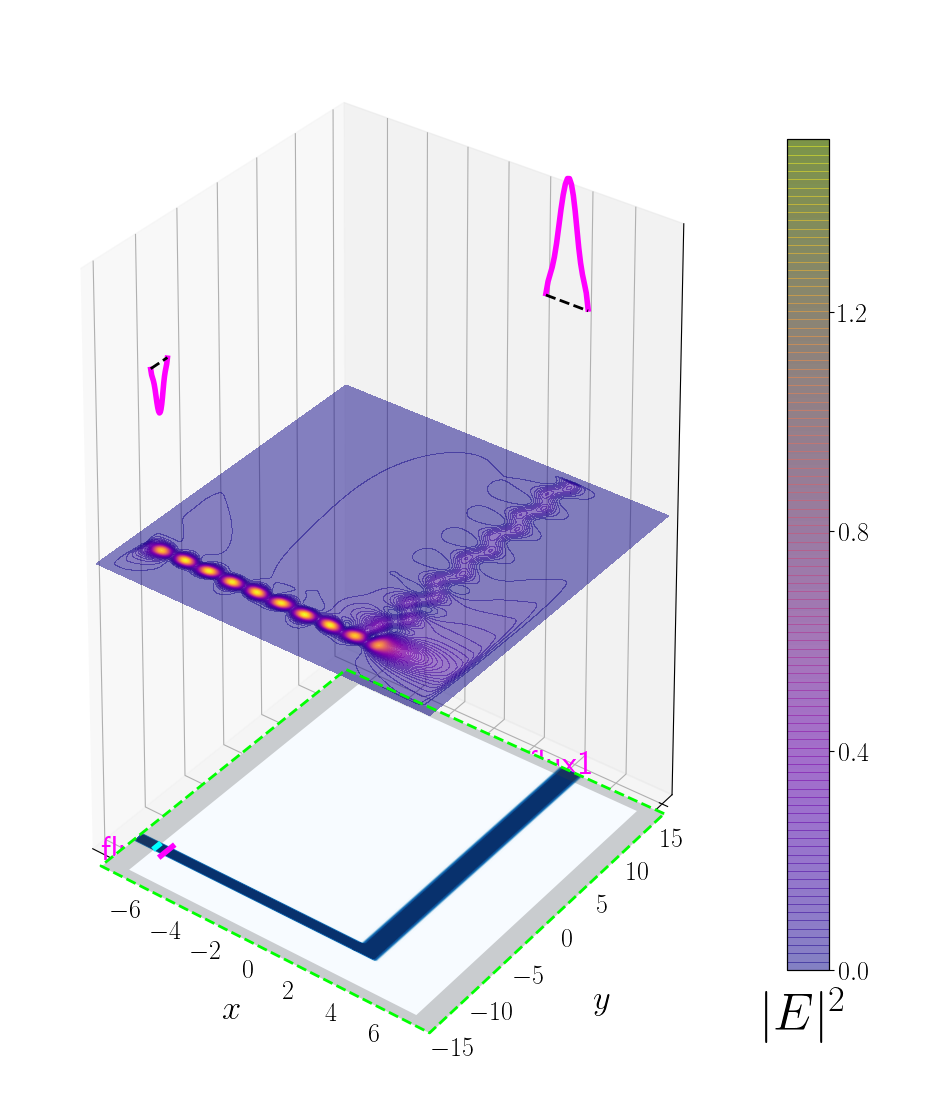Meep contains an adjoint solver for efficiently computing the gradient of an objective function with respect to the permittivity on a discrete spatial grid in a subregion of the cell. Regardless of the number of degrees of freedom for the grid points, just two separate timestepping runs are required. The first run is the "forward" calculation to compute the objective function. The second run is the "adjoint" calculation which involves a special type of source distribution and postprocessing applied to the results. This module is implemented entirely in Python and does not involve modifications to the C++ libmeep core library. At a higher level, the module implements functionality for wrapping a numerical optimizer around the gradient computation to enable automated design optimization.

The procedure for using the adjoint solver is described below. To skip to a demonstration, see the example for a cross router; the script is in examples/adjoint_optimization/CrossRouter.py.

## Setting Up the Adjoint Calculation

There are two components to setting up an adjoint calculation in Meep:

1. Implement a subclass of the OptimizationProblem virtual base class defined by meep.adjoint. This subclass must provide overrides for the virtual class methods init_problem and create_sim.

2. Execute the Python script with command-line options specifying which of various calculations to perform ranging in complexity from (a) evaluating an objective function and its gradient for a single design to (b) iterative optimization in which the design is repeatedly evaluated and tweaked automatically based on the gradient computation.

The init_problem method inputs an args structure containing the parsed values of command-line options, performs any necessary internal initialization, and returns a five-tuple describing the optimization problem:

• fstr: string specifying your objective function
• objective_regions: list of regions over which to compute frequency-domain (DFT) fields needed to evaluate the quantities on which your objective function depends
• extra_regions: list of additional regions over which to compute DFT fields for post-processing or visualization (this is often empty)
• design_region: variable-permittivity region of your geometry
• basis: set of basis functions used to expand the permittivity. This is a subclass of the Basis base class defined by meep.adjoint; you may implement your own arbitrary basis, or use one of several predefined bases provided by meep.adjoint.

To add your own command-line options or specify values for the available options, you need to override the add_args method of OptimizationProblem. For a demonstration, refer to the examples in python/examples/adjoint_optimization.

The following are three examples of fstr strings for defining objective functions.

• Maximize the Poynting flux in the first DFT flux region of your objective_regions list:
fstr = 'S_0'

• Minimize the difference between the Poynting flux in the DFT flux regions labeled 'north' and 'south':
fstr = '-(S_north - S_south)^2'

• Maximize the squared magnitude of the eigenmode coefficient (i.e., the Poynting flux) for the forward-traveling component of eigenmode 3 at the 'east' flux region:
fstr = 'Abs2(P3_east)^2'


The basis field should be the predefined FiniteElementBasis class which describes a localized basis of functions over a rectangular domain with variable resolution:

basis=FiniteElementBasis(design_region_width, design_region_height, density=3)


The parameter density specifies the number of finite-element subdivisions per unit length.

The create_sim method is a subclass that must be overridden. The input is a vector beta_vector of basis expansion coefficients and returns a Simulation object describing your geometry with the corresponding spatially-varying permittivity in the design region. The simplest way to do this is to include in your geometry list an object whose spatial extent coincides with the design_region returned by your init_problem and whose epsilon_func is an instance of the ParameterizedDielectric class defined by meep.adjoint. See the examples in python/examples/adjoint_optimization.

## Running the Adjoint Calculation

If you want to be able to run your script as an executable from the shell, copy and paste the following boilerplate code verbatim into bottom of your script:

######################################################################
# if executed as a script, we look at our own filename to figure out
# the name of the class above, create an instance of this class called
# op, and call its run() method.
######################################################################
if __name__ == '__main__':
op=globals()[__file__.split('/')[-1].split('.')]()
op.run()


Once the Python script with subclass OptimizationProblem has been created, it can be executed with a number of command-line options. The following are several examples.

• plot the geometry with all options set to default values:
% python MyOptimizationProblem.py

• plot the geometry with a given functional form for the initial design permittivity:
% python MyOptimizationProblem.py --eps_design '1.0+5.0*(1.0+sin(x)*cos(2.0*y))'

• compute the objective function at the initial design point:
% python MyOptimizationProblem.py --eval_objective

• compute the objective function and gradient at the initial design point:
% python MyOptimizationProblem.py --eval_objective

• run the full iterative optimization:
% python MyOptimizationProblem.py --optimize


The full list of command-line options is documented here.

### Running Jobs in Parallel

Aside from the adjoint solver, the meep.adjoint module provides functionality for embarrassingly parallel workloads. The standalone module meep.adjoint.ParallelDesignTester offers a simple way to parallelize a large number of runs of a Python script with different command-line options. Thus, suppose you want to run the script MyScript.py with 100 different sets of command-line options, i.e. you want to achieve the effect of running the following sequence of shell commands:

% python MyScript.py '--x 1.0 --name x1'
% python MyScript.py '--x 2.0 --name x1'
% ...
% ...
% ...
% python MyScript.py '--x 100.0 --name x100'


The following script will launch a pool of N processes running N jobs thereby yielding an N-fold speedup:

from meep.adjoint import ParallelDesignTester

cmdlines=[
'--x 0 1.0 --name x1',
'--x 0 2.0 --name x2',
...
...
...
'--x 0 100.0 --name x100']

ParallelDesignTester(MyScript,cmdlines).launch()


The default number N of server processes launched is half the number of CPU cores on your machine, but may be set to e.g. 4 via launch(nproc=4).

Additionally, the meep.adjoint.Visualization module provides routines for visualization. For example, the function visualize_sim() when called after initializing the Simulation object and just before timestepping displays the geometry, PML layers, source regions, and DFT regions.

from meep.adjoint import visualize_sim

sim=mp.Simulation(...)

# code to add DFT cells goes here

visualize_sim(sim)


## Visualizing Simulation Results: Frequency-Domain Fields

You can call visualize_sim() after timestepping to visualize the DFT fields superimposed over the geometry:

sim.run(...)
visualize_sim(sim)


This routine is designed for quick-and-easy visualization of the geometry without the need to configure a bunch of customization options. For example, the following is its output before and after timestepping for Tutorial/Basics/A 90° Bend.

Before Timestepping:After Timestepping:See the adjoint-solver documentation for more examples of pre- and post-timestepping visualizations generated by meep.adjoint.Visualization.

Note: Although visualize_sim is designed to do something useful on any geometry with no command-line options, it also offers numerous options for customizing the visualization. For details, refer to the documentation.

## Real-Time Visualization of Time-Domain Fields

The visualization module also provides functionality for real-time visualization of the time-domain fields. For example, the module provides a step function AFEClient which intermittently updates a plot of the field profile during time stepping. More specifically, AFEClient is a class defined in meep.adjoint that accepts initialization options and may then subsequently be called as a step function via its __call__ method. Behind the scenes, it implements the client side of a client-server visualization protocol in which the heavy lifting of the visualization is done by a separate process to offload its computational burden from the Meep timestepping process. See the AFE_Client and AFE_Server functions python/adjoint/Visualization.py.

For example, to update a plot of Ez every two timesteps involves:

step_funcs = [ AFEClient(sim, ['Ez'], interval=2) ]
sim.fun(*stepfuncs, until=200)


The following are two animations of the fields in the holey waveguide showing an incident eigenmode propagating across a waveguide with and without a hole in it.